# How To Division Formula in Excel

Feb 01, 2020 • edited Feb 02, 2020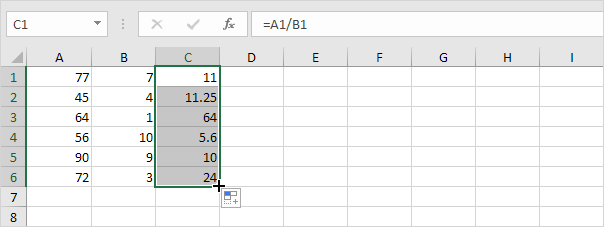Excel doesn't have a divide function, so performing division in Excel requires you to create a formula. Let's learn how to use formulas to divide numbers and calculate percentages in Excel.

dividing in Excel is easy, but you need to create a simple formula to do it. Just remember that all formulas in Excel begin with an equal sign (=), and you can use the formula bar to create them.

Division in Excel

Here are some important points to remember about Excel formulas:

• Formulas begin with the equal sign ( = ).
• The equal sign goes in the cell where you want the answer to display.
• The division symbol is the forward slash ( / ).
• The formula is completed by pressing the Enter key on the keyboard.

1. The formula below divides numbers in a cell. Use the forward slash (/) as the division operator. Don't forget, always start a formula with an equal sign (=).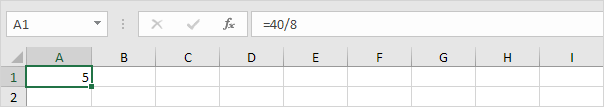2. The formula below divides the value in cell A1 by the value in cell B1.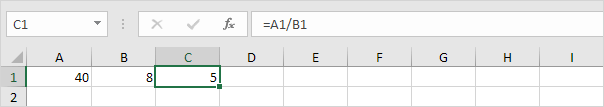3. Excel displays the #DIV/0! error when a formula tries to divide a number by 0 or an empty cell.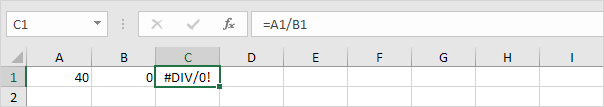4. The formula below divides 43 by 8. Nothing special.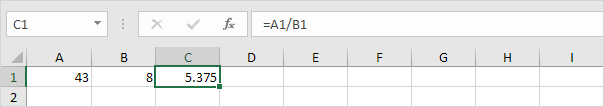5. You can use the QUOTIENT function in Excel to return the integer portion of a division. This function discards the remainder of a division.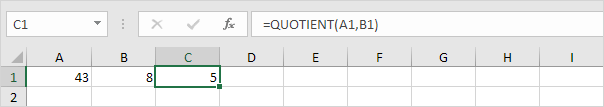6. The MOD function in Excel returns the remainder of a division.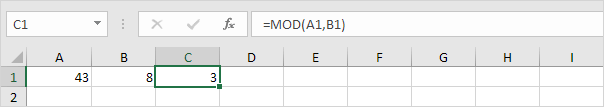Take a look at the screenshot below. To divide the numbers in one column by the numbers in another column, execute the following steps.

7a. First, divide the value in cell A1 by the value in cell B1.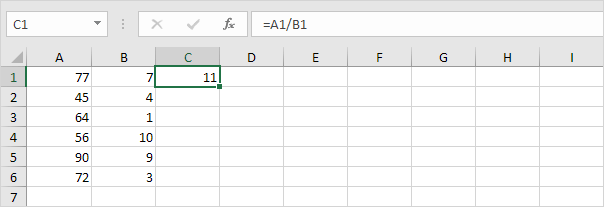7b. Next, select cell C1, click on the lower right corner of cell C1 and drag it down to cell C6.Take a look at the screenshot below. To divide a column of numbers by a constant number, execute the following steps.

8a. First, divide the value in cell A1 by the value in cell A8. Fix the reference to cell A8 by placing a \$ symbol in front of the column letter and row number (\$A\$8).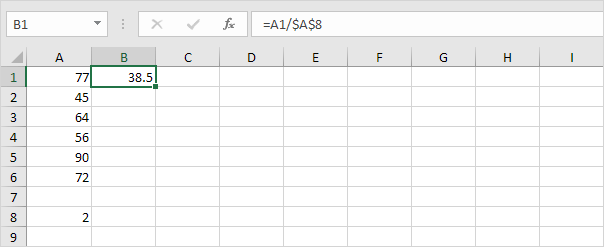8b. Next, select cell B1, click on the lower right corner of cell B1 and drag it down to cell B6.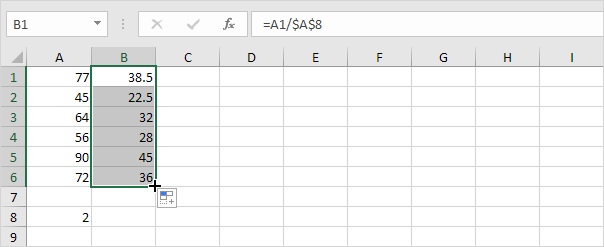Explanation: when we drag the formula down, the absolute reference (\$A\$8) stays the same, while the relative reference (A1) changes to A2, A3, A4, etc.

#How To#Tutorial#Formulas#Functions

How to use Square Root in Excel

How to Multiply and divide numbers in Excel